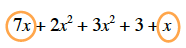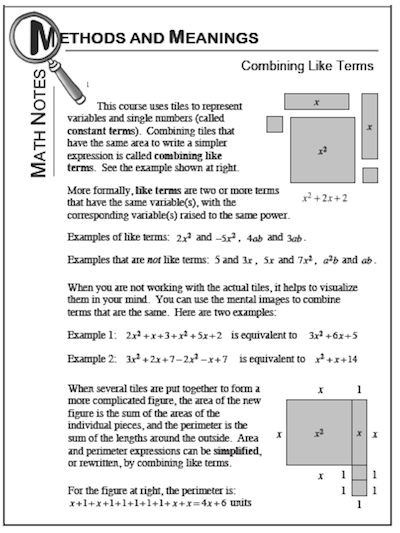### Home > CC1 > Chapter 6 > Lesson 6.2.4 > Problem6-107

6-107.

Sketch the collection of algebra tiles that is described by the following expression. Rewrite the area of the collection by combining like terms. Homework Help ✎

$7x+2x^2+3x^2+3+x$

How many total x-tiles are there?

Circle any term that has only one x in it.
Then add the coefficients.Note: A variable without a coefficient showing actually has a coefficient of 1.

For more help, refer to the Math Notes box.